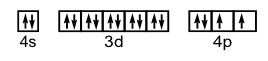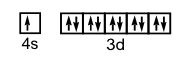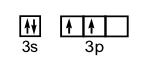Chapter 8. Electronic Structure of Atoms

# 8.6 End of Chapter Problems

1. Calculate the frequency and wavelength of light that is emitted contains 3.45 x 10-18J of energy.  What type of light is this?

2. Convert the following light wavelengths into frequencies (Hz). (The speed of light c=2.998 x 108m/s)

a)  4.33 nm          b) 2.35 x 10-10m          c)  735 nm          d) 4.57 mm

3. Convert the following light frequencies into wavelengths (expressing the result in the indicated units), assuming the light is moving at the speed of light (c=2.998 x 108m/s)

a)  4.77 GHz (m)          b) 2.89 kHz (cm)

c)  50. Hz (mm)          d) 2.88 MHz (mm)

4. How does the energy possessed by an emitted photon compare to the difference in energy levels that gave rise to the emission of the photon?

5. Explain the difference between continuous and discrete spectra.

6. Describe one aspect of Bohr’s model of the atom that “worked”. Describe one aspect of Bohr’s model of the atom that did not “work”.

7. Draw an electron shell model of the following

a)  chloride ion, Cl1-          b) calcium ion, Ca2+

8. In what way are outer electron shells of oxygen and selenium similar? In what way are they different?

9. Why is the view of an electron being a particlein a fixed orbitunacceptable?

10. Explain the difference between an orbit and an orbital. Briefly describe the model that each refers to.

11. Which law(s) (e.g.: Aufbau principle, Pauli exclusion principle or Hund’s rule) is (are) violated in each of these ground state electron configurations. Write the correct configuration as well.

a)  B = 1s12s12px12py12pz1

b)  Sc = [Ne] 3s23p63d3

c)  Na = 1s21p72s23s1

d)  C = 1s22s22px2

12. Write the electron configurations and show the orbital box diagram for each of the following. Show only the valence electrons with the box notation.
a) Se      b) Cu     c) Fe    d) Si

13. Write the electron configuration, using core notation (condensed electron configuration), for the following.
a) Pt      b) Zr      c) W

14. How many orbitals in an atom can have the designation: 5p, 4d, n=5, n=4?

15. The elements Si, Ga, As, Ge, Al, Cd, S and Se are all used in the manufacturing of various semiconductor devices. Write the expected electron configuration for each of these atoms

16. Write the expected ground-state electron configuration for the following:

a) The element(s) with one unpaired 5p electron.

b) The, as yet undiscovered alkaline earth metal after (i.e. below in the periodic table) radium.

c) The noble gas with electrons occupying 4f orbitals.

d) The first-row transition metal with the most UNPAIRED electrons (i.e. electrons singly in orbitals).

17. Which of the following electron configurations correspond to an excited state (i.e., is not a ground-state/lowest energy electron configuration)?  Identify the atoms and write the ground-state electron configurations where appropriate.

a)  1s22s23p1                                                 b)  1s22s22p6
c)  1s22s22p43s1                                         d) [Ar]4s23d54p1

18. Arrange the following in order of increasing atomic size:  N, O, S

19. Arrange the following in order of increasing ionization energy:  S, P, F

20. How many valence electrons do the following atoms have?

a)  Li           b) Al           c)  P

21. Arrange the following groups of atoms in order of increasing size:

a)  Be, Mg, Ca                   b)  Te, I, Xe                  c)  Ga, Ge, In

22. Arrange the atoms in the previous exercise in order of increasing first ionization energy.

23. In each of the following sets, which atom or ion has the smallest radius?

a)  Li, Na, K        b) P, As        c)  O+, O, O        d) S, Cl, Kr        e)  Pd, Ni, Cu

24. The first ionization energies of As and Se are 0.947 and 0.941 MJ/mol respectively.  Rationalize these values in terms of electron configurations.

25. For each of the pairs of elements  (O and F) and  (Ar and Br), pick the atom with:

a)  higher (more negative) electron affinity
b)  higher ionization energy
c)  larger size

26. What is the frequency of light if its wavelength is 1.00 m?

27. What is the energy of a photon if its wavelength is 1.00 meter?

28.  a)  Predict the electron configurations of Sc through Zn.

b)  From a source of actual electron configurations, determine how many exceptions there are from your predictions in part a.

29.  Recently, Russian chemists reported experimental evidence of element 117. Use the periodic table to predict its valence shell electron configuration.

30.  Which atom has a higher ionization energy (IE), O or P?

31.  Which atom has a smaller radius, As or Cl?

32.  How many IEs does an H atom have? Write the chemical reactions for the successive ionizations.

33.  Based on what you know of electrical charges, do you expect Na+ to be larger or smaller than Na?

1.   5.21 x 1015s-1;   5.76 x 10-8m;   UV

2.   a) 6.92 x 1016Hz       b) 1.28 x 1018Hz      c) 4.08 x 1014Hz      d) 6.56 x 1013Hz

3.   a) 0.0629 m      b) 1.04 x 107cm      c) 6.0 x 109mm      d) 1.04 x 108um

4.   equal

5.   continuous spectra contain all frequencies while discrete spectra only contain limited number of frequencies.

6.   The concept that the energy of the electron is quantized is true. The concept that the electron travels on a fixed path or “orbit” is not the case.

7.   a)                                              b)8.   They are similar in that they both need 2 more electrons to have a full outer shell. They are different in that they have a different number of electrons in the outer shell. O has 6 but Se has16.

9.   Viewing the electron as a particle does not recognize that it has wave-like properties. Having a fixed orbit violates the Uncertainty Principle.

10.  orbit: 2-D circular path in which an electron can be found. Orbital: 3-D region of space in which there’s a high probability of finding the electron.

11.  a) Aufbau. 1s22s22p1                                            b) Aufbau. [Ar]4s23d1
c) Aufbau & Pauli exclusion. 1s22s22p63s1         d) Hund’s rule. 1s22s22p2

12. Note: only the valence box diagram is shown in the following answers

a)  1s22s22p63s23p64s23d104p4b)  1s22s22p63s23p64s13d10c)  1s22s22p63s23p64s23d6d)  1s22s22p63s23p213.  a) [Xe]6s14f145d9
b)  [Kr]5s24d2
c)  [Xe]6s24f145d4

14.  3; 5;  25;  16

15.  Si: 1s22s22p63s23p2
Ga: 1s22s22p63s23p64s23d104p1
As: 1s22s22p63s23p64s23d104p3
Ge: 1s22s22p63s23p64s23d104p2
Al: 1s22s22p63s23p1
Cd: 1s22s22p63s23p64s23d104p65s24d10
S: 1s22s22p63s23p4
Se: 1s22s22p63s23p64s23d104p4

16.  a) In: [Kr]5s24d105p1or I: [Kr]5s24d105p5
b) Z = 120: [Rn]7s25f146d107p68s2
c) Rn: [Xe]
6s24f145d106p6
d) Cr: [Ar]4s13d5

17.  a) excited state of B; 1s22s22p1
b) ground state of Ne
c) excited state of F: 1s22s22p5
d) excited state of Fe: [Ar]4s23d6

18O < N < S

19.  P < S < F

20.  a) 1;    b) 3;    c) 5

21.  a) Be < Mg < Ca
b) Xe < I < Te
c) Ge < Ga < In

22.  a) Ca < Mg < Be
b) Te < I < Xe
c) In < Ga < Ge

23.  a) Li;  b) P;   c) O+;   d) Cl;  e) Cu

24.  As: [Ar]4s23d104p3and Se: [Ar]4s23d104p4. As has a half-filled 4p orbital which is more stable; Se has 2e in one of its 4p orbitals which gives rise to a larger electron-electron repulsion, making it easier for Se to lose an electron to reach a more stable half-filled 4p configuration, therefore Se has a smaller IE1.

25.  a)  F, Br;    b) F, Ar;    c) O, Br

26. 3.00 × 108 s−1

27. 1.99 × 10−22 J

28. a)  The electron configurations are predicted to end in 3d1, 3d2, 3d3, 3d4, 3d5, 3d6, 3d7, 3d8, 3d9, and 3d10.

b)  Cr and Cu are exceptions.

29. Element 117’s valence shell electron configuration should be 7s27p5.

30. O

31. Cl

32. H has only one IE: H → H+ + e

33. smaller